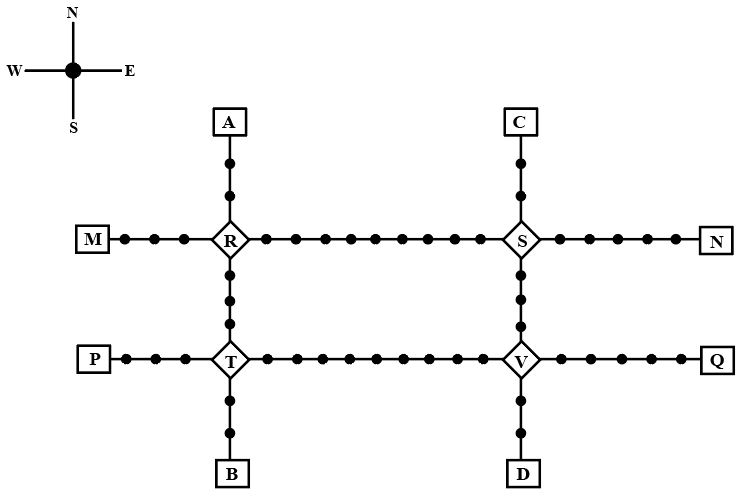### CAT 2022 Question Paper (Slot 1) Question 43

InstructionsGiven above is the schematic map of the metro lines in a city with rectangles denoting terminal stations (e.g. A), diamonds denoting junction stations (e.g. R) and small filled-up circles denoting other stations. Each train runs either in east-west or north-south direction, but not both. All trains stop for 2 minutes at each of the junction stations on the way and for 1 minute at each of the other stations. It takes 2 minutes to reach the next station for trains going in east-west direction and 3 minutes to reach the next station for trains going in northsouth direction. From each terminal station, the first train starts at 6 am; the last trains leave the terminal stations at midnight. Otherwise, during the service hours, there are metro service every 15 minutes in the north-south lines and every 10 minutes in the east-west lines. A train must rest for at least 15 minutes after completing a trip at the terminal station, before it can undertake the next trip in the reverse direction. (All questions are related to this metro service only. Assume that if someone reaches a station exactly at the time a train is supposed to leave, (s)he can catch that train.)

Question 43

# What is the minimum number of trains that are required to provide the service on the AB line (considering both north and south directions)?

Solution

Travel time between A and B = $$\left(10\times3\right)+\left(7\times1\right)+\left(2\times2\right)=41$$ minutes

After completing a journey, a train must rest for 15 minutes at least before starting again.

So if a train starts from 6 am from A to B, then the latest by which that train will start from B to A will be at 7 am, as in the north-south direction, a train starts from A and B every 15 minutes.

So the total no. of trains required = $$\left(\frac{60}{15}\right)\times2=8$$